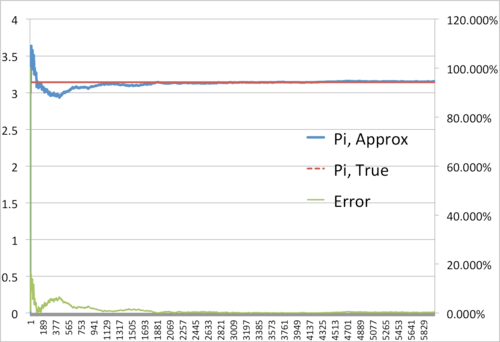# Monte Carlo Simulation of Pi

Partially out of boredom and partially because I was inspired by the movie title “Life of Pi”, I decided to make a monte carlo simulator that could approximate the value of pi.

Monte carlo simulations are used in everything from derivative pricing to biology (and, in this case, boredom alleviation). Basically, it’s good for solving problems that have no exact solution.

The simulator throws a random point on a 2×2 square and then throws a random point on a circle of radius 1. This is one trial. However, it does this (in this case) for 6000 trials. So the square is filled with 6000 points and the circle is filled with less than 6000 points.

Now that the simulation has done a really good job at filling in the square and the circle, we count the number of points in the the circle and the square to get the areas of each. Then we take the ratio: areaCir/areaSq.

However, we know that the areaSq is 4 for a 2×2 square. So we multiple that ratio by 4 to cancel out the denominator and are left with areaCir. Since areaCir=pi*radius^2, areaCir=pi for our circle because radius=1.

The more trials we conduct, the more filled our circle and square become and, thus, the more accurate out approximation of pi. Here is the result:It’s clear that as trials -> ∞ , error -> 0% and Pi,Approx -> Pi,True

Doing more than 6000 trials really slows down processing unless the simulator is done in code. So, I built a monte carlo simulator using VBA.

I ran 50,000,000 simulations. It took 34.2187 seconds and it approximated pi=3.14155704. Pi is actually equal to 3.14159265. Error was .001%